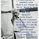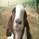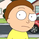6617 lượt xem
6617
Fixed the previous version which, when TradingView updated, resulted in an error.
A mid-line could be plotted with (hpivot+lpivot)/2

*Oops plotted some gann fans with the script, the script you are looking at is the faint lines in the background
study(title="Pivot Points H/L", shorttitle="Pivots H/L", overlay=true)
len = input(14, minval=1, title="Length")
//The length defines how many periods a high or low must hold to be a "relevant pivot"

h = highest(len)
//The highest high over the length
h1 = dev(h, len) ? na : h
//h1 is a pivot of h if it holds for the full length
hpivot = fixnan(h1)
//creates a series which is equal to the last pivot

l = lowest(len)
l1 = dev(l, len) ? na : l
lpivot = fixnan(l1)
//repeated for lows

plot(hpivot, color=blue, linewidth=2, offset= -len+1)
plot(lpivot, color=purple, linewidth=2, offset= -len+1)
//plot(h1, color=black, style=circles, linewidth=4, offset= -len+1)
//plot(l1, color=black, style=circles, linewidth=4, offset= -len+1)any way you could modify to include the mid-line - i'm not a programmer.

Thx!
Phản hồiucsgears
Can you program the PyraPoint? That would be awesome. Thx for this pivot script
Phản hồigreenplay
Add to Favorite Scripts at the top right of the code. If you were attracted by the gann fans you can get to those from the drawing tools, they're in the same section as the pitchfork.
Phản hồi